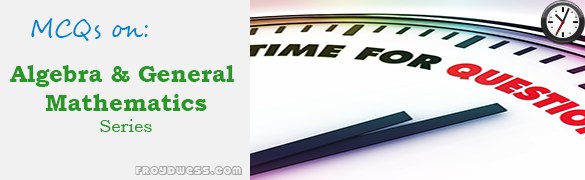# MCQ in Algebra and General Mathematics Series | Math Board ExamThis is the list of Multiple Choice Questions in Algebra and General Mathematics Series topics in Engineering Mathematics. In Preparation for the ECE Board Exam make sure to expose yourself and familiarize in each and every questions compiled here taken from various sources including but not limited to past Board Examination Questions in Engineering Mathematics, Mathematics Books, Journals and other Mathematics References.

#### MCQ Topic Outline included in Mathematics Board Exam Syllabi

• MCQ in Algebraic functions
• MCQ in theory of Equations
• MCQ in Factorization and Algebraic functions
• MCQ in Ratio, Proportion and Variation
• MCQ in Matrix theory
• MCQ in Arithmetic and Geometric Progression
• MCQ in Equations and Inequalities
• MCQ in Linear and Quadratic Equations
• MCQ in Complex Number System
• MCQ in Polynomials
• MCQ in Mathematical Induction
• MCQ in Logic and Probability
• MCQ in Statistics
• MCQ in System of Numbers and Conversion
• MCQ in Fundamentals in Algebra
• MCQ in Binomial Theorems and Logarithms
• MCQ in Age Problems
• MCQ in Work Problems
• MCQ in Mixture Problems
• MCQ in Digit Problems
• MCQ in Motion Problems
• MCQ in Clock Problems
• MCQ in Variation
• MCQ in Progression
• MCQ in Miscellaneous Problems

### Online Questions and Answers in Algebra and General Mathematics Series

Following is the list of multiple choice questions in this brand new series:

MCQ in Algebra and General Mathematics
PART 1: MCQ from Number 1 – 50                               Answer key: PART 1
PART 2: MCQ from Number 51 – 100                          Answer key: PART 2
PART 3: MCQ from Number 101 – 150                        Answer key: PART 3
PART 4: MCQ from Number 151 – 200                         Answer key: PART 4
PART 5: MCQ from Number 201 – 250                         Answer key: PART 5
PART 6: MCQ from Number 251 – 300                         Answer key: PART 6
PART 7: MCQ from Number 301 – 350                         Answer key: PART 7
PART 8: MCQ from Number 351 – 400                         Answer key: PART 8
PART 9: MCQ from Number 401 – 450                         Answer key: PART 9
PART 10: MCQ from Number 451 – 500                        Answer key: PART 10
PART 11: MCQ from Number 501 – 550                        Answer key: PART 11

### Online Questions and Answers in Fundamentals in Algebra Series

Following is the list of practice exam test questions in this brand new series:

MCQ in Fundamentals in Algebra
PART 1: MCQ from Number 1 – 50                           Answer key: PART 1
PART 2: MCQ from Number 51 – 100                      Answer key: PART 2

PinoyBIX Engineering. © 2014-2020 All Rights Reserved | How to Donate? |